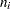FREQ Statement
FREQ variable ;

The FREQ statement names a variable that provides frequencies for each observation in the DATA= data set. Specifically, ifis the value of the FREQ variable for a given observation, then that observation is usedtimes.

The analysis produced by using a FREQ statement reflects the expanded number of observations. For example, means and total degrees of freedom reflect the expanded number of observations. You can produce the same analysis (without the FREQ statement) by first creating a new data set that contains the expanded number of observations. For example, if the value of the FREQ variable is 5 for the first observation, the first 5 observations in the new data set would be identical. Each observation in the old data set would be replicatedtimes in the new data set, whereis the value of the FREQ variable for that observation.

If the value of the FREQ variable is missing or is less than 1, the observation is not used in the analysis. If the value is not an integer, only the integer portion is used.

If the FREQ statement is used, it must appear before the first RUN statement or it is ignored.